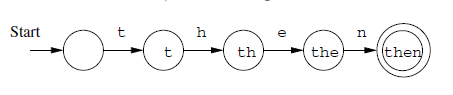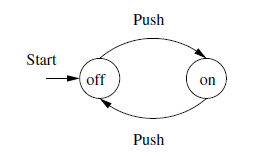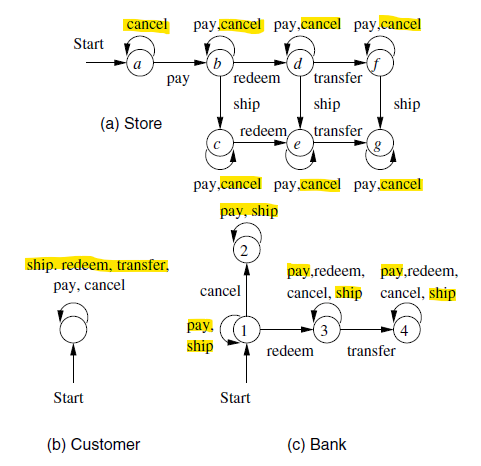# Automata - State

The state page in automata.

## Syntax

### Set

Q is the symbol for the set of states

### Start

q is the symbol for the start state.

### State after a serie of transitions

The state that the automaton $A$ is in after processing input $w$ is expressed by the below expression: $$\delta(A,w)$$

where: $\delta$ is a symbol for a transition function

## Property

in one of this state, the automaton will operate:

• final
• accepting

Entering one of these states after a sequence of inputs indicates that the input sequence is good in some way

It is conventional to designate accepting states by a double circle

Discover MoreAutomata - Deterministic finite-state automata (DFA)

A Deterministic finite-state automata (DFA) is a finite automaton that cannot be in more than one state at any one time. The term deterministic refers to the fact that on each input there is one and only...Automata - Finite Automata

A finite automaton is an automaton that has a set of states and its control moves from state to state in response to external inputs. It has a start and an end state and there are only a finite number...Automata - Transition

In a automata, a Transition is the fact of going from one state to another and is represent by a edge in a graph. It's a function that: takes as arguments a state and an input symbol (The transition...Automaton (State Machine)

Automaton (State Machine) Automata theory is the study of abstract computing devices or machines. The word automata (the plural of automaton) comes from the Greek word αὐτόματα, which means...Data - State

State is the attribute values of a thing at a given moment. A State can be seen as a snapshot of values at a precise moment. entityattributestatusa lifecyle Edson...Data Processing - (Pipeline | Compose | Chain)

A pipeline is a finite automata where: the data transition from one state to another via a series of transformations (work) A pipeline creates a composition relationship. A pipeline is also...Process - LifeCycle (Entity State) (Status)

In a process based model, every entity has a lifecycle (Status) that shows a finite number of distinct states. The status shows the state of an entity ie: finite automata See also: Sequence:...What is a Lexer ? known also as Tokenizer or Scanner - Lexical Analysis

Lexer are also known as: * Lexical analyzer * Lexical Tokenizer * Lexical Scanner Consider the following expression: A lexer will tokenized it in the following symbol table: Token Lexeme ...